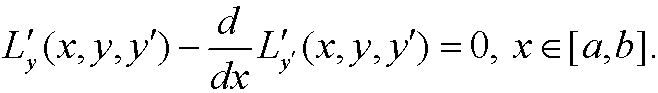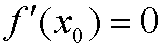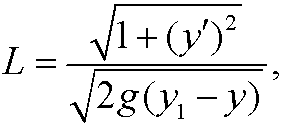# 5. Euler's equation for the simplest problem.

Theorem 1: If a functiony  is extremal to the functionwherey belongs to C2[a,b] andthen y  must fulfill the Euler equationRemark: The Euler equation corresponds to the conditionin extreme value problems and is an ordinary differential equation that in general is nonlinear. By using the chain rule, we see that it can be expanded asThe function L=L(x,y,y) is called the Lagrangian of the problem. In our previous problems problems 45 and 6  the Lagrangians wereandrespectively.